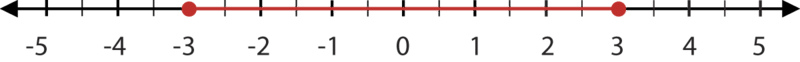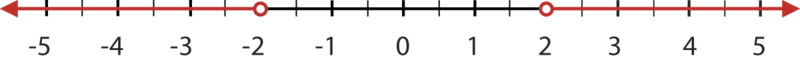# 3.10: Graph and Solve Absolute Value Inequalities

•• Contributed by CK12
• CK12

## Absolute Value Inequalities

Suppose the perimeter of a square garden is currently 4l meters, where l is the length of one of its sides, and that you want to change the perimeter to 32 meters. However, you don't want to change the perimeter by more than 8 meters. What must the length of one of its sides currently be?

### Absolute Value Inequalities

An absolute value inequality is a combination of two concepts: absolute values and linear inequalities. Therefore, to solve an absolute value inequality, you must use the problem-solving methods of each concept.

• To solve an absolute value equation, use the definition.
• If d=|x−a|, then x−a=d OR x−a=−d.
• To solve a linear inequality, use the information learned in the previous Concepts.
• Remember when dividing by a negative the inequality symbol must be reversed.

#### Let's solve the following absolute value inequalities:

1. |x|≤3

Since |x| represents the distance from zero, the solutions to this inequality are those numbers whose distance from zero is less than or equal to 3. The following graph shows this solution:Notice that this is also the graph for the compound inequality −3≤x≤3.

1. |x|>2

Since the absolute value of x represents the distance from zero, the solutions to this inequality are those numbers whose distances from zero are more than 2. The following graph shows this solution.#### Forms of Solutions

In general, the solutions to absolute value inequalities take two forms:

1. If |x|<a, then x<a and x>−a.
2. If |x|>a, then x>a or x<−a.

#### Let's solve the following inequality using the forms from above:

Solve |x+5|>7.

This equation fits situation 2. Therefore, x+5>7 OR x+5<−7.

Solve each inequality separately.

x+5>7 x+5<−7

x>2 x<−12

The solutions are all values greater than two or less than –12.

### Examples

Example 3.10.1

Earlier, you were told that the perimeter of a square garden is currently 4l, where l is the length of one of its sides. You want to change the perimeter to 32 meters but you don't want to change the perimeter by more than 8 meters. What is the current length of one of the sides of the garden?

Solution

Since you do not want to change the perimeter by more than 8 meters, the distance between the current perimeter and 32 must be less than or equal to 8. The inequality that represents this situation is:

|4l−32|≤8

This is an example like case 1 from above. Separate and solve.

4l−32≤8 and 4l−32≥−8

4l≤40 and 4l≥24

l≤10 and l≥6

The solution to the inequality is 6≤l≤10. Therefore, the garden's current side length must be between 6 and 10, including both 6 and 10.

Example 3.10.2

The velocity of an object is given by the formula v=25t−80, where the time is expressed in seconds, and the velocity is expressed in feet per second. Find the times when the velocity is greater than or equal to 60 feet per second.

Solution

We want to find the times when the velocity is greater than or equal to 60 feet per second. Using the formula for velocity, v=25t−80, and substituting the appropriate values, we obtain the absolute value inequality |25t−80|≥60.

This is an example like case 2. Separate and solve.

25t−80≥60 or 25t−80≤−60

25t≥140 or 25t≤20

t≥5.6 or t≤0.8

### Review

1. You are asked to solve |a+1|≤4. What two inequalities does this separate into?

In 2–21, solve each inequality and show the solution graph.

1. |x|≤6
2. 4≤|a+4|
3. |x|>3.5
4. 6>|10b+6|
5. |x|<12
6. ∣w/10∣<2
7. ∣x5∣≤6
8. |7x|≥21
9. |6c+5|<47
10. |x−5|>8
11. |x+7|<3
12. |x−(3/4)|≤12
13. |2x−5|≥13
14. |5x+3|<7
15. ∣(x/3)−4∣≤2
16. ∣(2x/7)+9∣>5/7
17. |−6t+3|+9≥18
18. |9p+5|>23
19. |−2s−4|≤6
20. |10m−5|/8>5
21. A three-month-old baby boy weighs an average of 13 pounds. He is considered healthy if he is 2.5 pounds more or less than the average weight. Find the weight range that is considered healthy for three-month-old boys.

### Vocabulary

Term Definition
absolute value If d=|x−a|, then x−a=d OR x−a=−d.
Absolute Value Linear inequalities Absolute value linear inequalities can have one of four forms |ax+b|>c,|ax+b|<c,|ax+b|≥c, or |ax+b|≤c. Absolute value linear inequalities have two related inequalities. For example for |ax+b|>c, the two related inequalities are ax+b>c and ax+b<−c.

PLIX: Play, Learn, Interact, eXplore: Absolute Value Inequalities: The Nuts and Bolts of Allowable Error

Video: Graphing Absolute Value Inequalities - Overview

Activity: Absolute Value Inequalities Discussion Questions

Study Aid: Absolute Value Equations and Inequalities Study Guide

Practice: Graph and Solve Absolute Value Inequalities

Real World Application: Body Temperature